# Convective Instability in Binary Nanofluids for Absorption Phenomenon with Cross Diffusions and Internal Heat Source

Convective Instability in Binary Nanofluids for Absorption Phenomenon with Cross Diffusions and Internal Heat Source
Department of Mathematics, Gulbarga University, Kalaburagi 585 106, India

Corresponding Author Email:
Page:
1047-1056
|
DOI:
https://doi.org/10.18280/ijht.390402
29 December 2020
|
Accepted:
2 August 2021
|
Published:
31 August 2021
| Citation

OPEN ACCESS

Abstract:

Theoretical investigation of cross diffusion effects on the convective stability of binary nanofluids under the influence of internal heat source is carried out using linear stability theory and addition factor analysis. Cross diffusions include Soret and Dufour effects. Soret effect implies that mass diffusion induced by thermal gradient and Dufour effect implies that heat transfer induced by concentration gradient. The physical system containing a binary nanofluid of rigid, impermeable to mass flow and perfectly thermal conducting boundaries where temperatures and concentrations are constant but different is taken. The stability equations are framed and the formulation of Rayleigh numbers is made for stationary and oscillatory states of the system. The effects of dimensionless parameters like internal Rayleigh number, Soret coefficients of nanoparticles and solute, volume fraction of nanoparticles and Dufour coefficient on the system are represented graphically. Ammonia/water based silver and alumina, the two binary nanofluids are considered and impact of nanoparticles through the addition factor on stability of the system is discussed pictorially. It is observed that the internal Rayleigh number reduces the size of convection cells. It causes to destabilize the system and improves the heat and concentration transfer in the system. Stability of the system under the influence of cross diffusion depends upoun the Soret effects of solute and nanoparticles. The Soret parameter and volume fraction of nanoparticles enlarge the size of convection cells and Dufour parameter reduces the size of convection cells in stationary state of the system. The reversed effects are reported in case of oscillatory state. The volume fraction of nanoparticles has destabilizing effect and it causes to increase the heat transfer in binary nanofluid.

Keywords:

binary nanofluid, convection, cross diffusions, Dufour effect, heat generation, separation ratio, thermophoresis

1. Introduction

Nanofluid, a fluid prepared by disseminating the nanometer-sized particles of metals, metallic oxides, carbides or carbon nanotubes steadily and consistently in regular host fluids like ethylene glycol, oil, water, etc. For the past decades, it plays a vital role in many fields owing to its extremely intensify thermal properties and significant applications as heat transfer fluids, ferromagnetic fluids, superwetting fluids and detergents, biomedical fluids, polymer nanocomposites, gain media in random lasers, building blocks for electronics and optoelectronics devices, etc. [1, 2]. Choi and Eastman  were the first, who proposed the term ‘nanofluid’. Many industrial processes like air conditioning and refrigeration, automobiles, distillation of solar water, foods freezing, etc. [4-6] are based on absorption phenomenon. In these processes, the binary mixture of fluids such as Ammonia - Ethelyne glycol, Ammonia - Sodium bromide, Ammonia - Water, Lithium bromide - Water, Carbon dioxide - Water, etc. are used instead of single fluids as host fluids. The binary nanofluids are the nanofluids in which the host fluids are such binary mixtures . The experimental observation on the enhancement of heat and mass transfer in binary nanofluids for absorption processes has been done by Ma et al. , Lee et al.  and Pang et al. . They have shown that the effect of shape and size of nanoparticles is more significant for enhancement of heat and mass transfer in binary nanofluids. It is studied by Xuan  theoretically and reported that the micro motion and stirring of suspended nanoparticles enhance the mass transport process in binary nanofluids. Such enhancement of mass diffusion in binary nanofluids depends on the size, volume fraction and state of motion of nanoparticles.

The convective instability in nanofluids has recently received considerable attention due to its numerous applications in many engineering phenomena such as chemical engineering, geothermal energy utilization, oil reservoir modeling, building of thermal insulation, solar energy, lubrication and biological processes [12, 13]. The convective stability problems in binary nanofluids using Buongiorno’s transport model  have been studied by many researchers for various situations. Few of the examinations are Nield and Kuznetsov , Yadav et al. , Bhadauria et al.  and Gupta et al. . The study on convective instability driven by buoyancy have been carried out by Kim et al.  by using an addition factor analysis and reported that the effect of addition of nanoparticles to the basefluid is to set in convective motion easily in nanofluid layer. Further, the convective stability in binary nanofluids for absorption phenomena with thermodiffusion and diffusiothermal effect of nanoparticles is studied theoretically by Kim et al. [20, 21]. They have shown that both effects decrease the stability of system of nanofluid and increase the transfer of heat more significantly.

Since few decades, the convective heat and mass transfer due to internal heating has got much attention because of its wide spread applications in the engineering problems related to energy like nuclear reactions, nuclear heat cores, nuclear energy, nuclear disposals, oil extractions, solar collectors, crystal growth, geophysical systems, etc. [22, 23]. The common phenomena of convections in nature induced by internal heat source include a motion in the atmosphere where heat is generated by absorption of sunlight and mantle convection in the earth where heat is generated by the radioactive decay of isotopes. Internal heating is the main source of convective heat and mass transfer caused by temperature gradient of the system. It keeps the system warm and active. Yadav et al.  were the first, who analysed the effect of internal heat source on stability of nanofluid layer with Brownian diffusion and thermophoresis of nanoparticles. It has been reported that the internal heat generating nanofluid layer increases the onset of convection in the system. The convection in an internally heated nanofluid layer considering the different boundaries as free-free, rigid-free and rigid-rigid is studied by Nield and Kuznetsov , and shown that the combinations of modified diffusivity ratio with nanoparticle Rayleigh number and Lewis number lead to make the nanofluid layer more unstable. Very recently, the effect of internal heating on stability of Rayleigh-Benard convection in different water-based nanoliquids and heat transfer enhancement of the nanoliquids through different shapes of enclosures has analysed by Kanchana and Zhao . Gaikwad and Surwase  have studied the convection phenomena in binary nanofluids with Soret effects and internal heat generation.

Keeping in view of the literature cited above, it seems that the problems of convective stability of binary nanofluids are of more interesting in the fields based on absorption phenomenon. However, theoretical work on this is rare. So, this motivated to do the present study with cross diffusion effects by carrying out addition factor analysis under the influence of internal heat source.

2. Problem Formulation

A horizontal layer of incompressible binary nanofluid which is at rest and lying in the region 0 ≤ z h is considered as shown in the Figure 1. The x-axis and z-axis are taken along horizontal and vertical directions respectively. The acceleration due to gravity is acting in the negative direction of z-axis. The boundaries of the layer are taken to be rigid, impermeable to mass flow and perfectly thermal conducting where temperatures and concentrations are constant but different. At the lower boundary z = 0, temperatures and concentrations are T0 + ∆T and C0 + ∆C respectively and at the upper boundary z = h, temperatures and concentrations are T0 and C0 respectively. When the binary nanofluid layer is heated from below, the buoyancy driven convection (Rayleigh – Benard convection) caused by temperature and concentration difference sets in over a certain thermal gradient.

2.1 Governing equations

The governing equations employing the Boussinesq approximations to study the convection in binary nanofluid under the influence of internal heat source with cross diffusions in dimensional form [21, 24, 27] are:

$\nabla \cdot \bar{q}=0$     (1)

${{\rho }_{0}}\left( \frac{\partial }{\partial t}\,\,\,+\,\,\,\bar{q}\cdot \,\nabla \right)\bar{q}=-\nabla P\,\,+\,\,\mu \,{{\nabla }^{2}}\bar{q}+\,\,\rho \bar{g}$     (2)

$\left( \frac{\partial }{\partial t}\,\,+\,\bar{q}\cdot \,\nabla \right)T=\alpha \,{{\nabla }^{2}}T+{{D}_{\text{d}\,\text{f}}}\,{{\nabla }^{2}}C+Q\,(T-{{T}_{0}})$     (3)

$\left( \frac{\partial }{\partial t}\,\,+\,\bar{q}\cdot \,\nabla \right)C=D\,{{\nabla }^{2}}C+{{D}_{\text{s}\,\text{r}}}\,{{\nabla }^{2}}T$     (4)

$\rho ={{\rho }_{0}}\left( 1-{{\beta }_{T}}(T-{{T}_{0}})+{{\beta }_{S}}(C-{{C}_{0}}) \right)$     (5)

The physical parameters involved in the Eqns. (1)-(5) have their typical sense and are prescribed in the nomenclature.

## 1.pngFigure 1. Geometrical configuration of physical system

2.2 Conduction state analysis

Employing the following time independent forms of the quantities: $\quad \bar{q}=\bar{q}_{b}=(u, v, w)=(0,0,0), P=P_{b}(z), T=$$T_{b}(z), C=C_{b}(z), \rho=\rho_{b}(z)$ and $\bar{g}=(0,0,-g)$ in Eqns. (1)-(5) the temperature and concentration at the basic state are given by:

${{T}_{b}}={{T}_{0}}+\Delta T\,\,\left( \frac{\sin \left( \sqrt{\frac{Ri}{1-\kappa }}\,\left( 1-\frac{z}{h} \right) \right)}{\sin \left( \sqrt{\frac{Ri}{1-\kappa }} \right)} \right)$     (6)

${{C}_{b}}={{C}_{0}}+\Delta C\left( 1-\frac{z}{h} \right)$      (7)

where, $\kappa =\frac{{{D}_{\text{s}\,\text{r}}}\,{{D}_{\text{df}}}}{\alpha \,D}$, and $Ri=\frac{Q\,{{h}^{2}}}{\alpha }$ is internal Rayleigh number.

3. Linear Stability Analysis

3.1 Perturbed state analysis

The infinitesimal disturbances are superposed on the quantities in conduction state:

$\bar{q}\,\,=\,\,{{\bar{q}}_{b}}\,+\,\,q',\,\,P\,\,=\,\,{{P}_{b}}\,\,+\,\,P',\,\,T\,\,=\,\,{{T}_{b}}\,\,+\,\,T',\,\,C\,\,=\,\,{{C}_{b}}+C',\,\,\rho \,\,=\,\,{{\rho }_{b}}\,\,+\,\,\rho ',$

the following equations are obtained:

$\nabla \cdot q'=0$     (8)

\begin{align} & \left( \frac{\partial }{\partial t'}+\,\,q'\cdot \nabla \right)q'=\,-\frac{1}{{{\rho }_{0}}}\,\nabla P'\,\,+ \\ & \,\,\,\,\,\,\,\,\,\,\,\,\,\,\,\,\,\,\,\,\,\,\,\,\,\,\,\,\,\,\,\,\,\,\,\,\,\,\,\,\,\,\,\,\,\,\,\,\,\,\,+\,\,\left( {{\beta }_{S}}C'-{{\beta }_{T}}T' \right)\bar{g}+\nu \,{{\nabla }^{2}}q' \\\end{align}      (9)

\begin{align} & \left( \frac{\partial }{\partial t'}+\,\,q'\cdot \nabla \right)T'+\left( \frac{\Delta T}{h}\,f(z) \right)w'=\,\, \\ & \,\,\,\,\,\,\,\,\,\,\,\,\,\,\,\,\,\,\,\,\,\,\,\,\,\,\,\,\,\,\,\,\,\,\,\,\,\,\,\,\,\,\,\,\,\,\,\,\,\,\alpha \,{{\nabla }^{2}}T'\,\,+{{D}_{\text{d}\,\text{f}}}\,{{\nabla }^{2}}C+Q\,T' \\\end{align}      (10)

\begin{align} & \left( \frac{\partial }{\partial t'}\,+\,\,q'\cdot \nabla \right)C'-\left( \frac{{{\beta }_{T}}\,{{C}_{s,i}}\,\Delta T}{h}\, \right)w'=\,D\,{{\nabla }^{2}}C'\, \\ & \,\,\,\,\,\,\,\,\,\,\,\,\,\,\,\,\,\,\,\,\,\,\,\,\,\,\,\,\,\,\,\,\,\,\,\,\,\,\,\,\,\,\,\,\,\,\,\,\,\,\,\,\,\,\,\,\,\,\,\,\,\,\,\,\,\,\,\,\,\,\,\,\,\,\,\,\,\,\,\,\,\,\,\,\,\,\,\,\,\,\,\,\,\,\,+{{D}_{\text{s}\,\text{r}}}{{\nabla }^{2}}T' \\\end{align}     (11)

where, q’, P’, T’ and C’ are the perturbed quantities.

Linearizing the Eqns. (9)-(11), introducing the nondimensional variables $\left( x,y,z \right)=\frac{1}{h}(x',y',z'),\,\,\,\,t=\frac{\alpha }{{{h}^{2}}}t',\,\,\,\,\bar{q}=\frac{h}{\alpha }q',\,\,T=\frac{T'}{\Delta T},\,\,C=\frac{C'}{\Delta C}$in the Eqns. (8)-(11) and then eliminating the pressure gradient term from reduced equation of Eq. (9), the following nondimensional equations are obtained:

\begin{align} & \left( \frac{1}{\Pr }\frac{\partial }{\partial t}-{{\nabla }^{2}} \right){{\nabla }^{2}}w\,\,=\,\,\left( \frac{{{\beta }_{s\,}}\Delta C\,{{h}^{3}}g}{\alpha \,\,\nu }\, \right)\,\,\nabla _{1}^{2}\,C \\ & \,\,\,\,\,\,\,\,\,\,\,\,\,\,\,\,\,\,\,\,\,\,\,\,\,\,\,\,\,\,\,\,\,\,\,\,\,\,\,\,\,\,\,\,\,\,\,\,\,\,\,\,\,\,\,\,\,\,\,\,\,\,\,\,\,\,\,\,\,\,\,\,\,\,\,\,\,-\,\,Ra\,\,\nabla _{1}^{2}\,T \\\end{align}      (12)

$\left( \frac{\partial }{\partial t}-\,\,{{\nabla }^{2}}\,-Ri \right)T=\,f(z)\,\,w\,\,+\,\,\left( \frac{{{D}_{\text{d}\,\text{f}}}\,\Delta C}{\alpha \,\Delta T} \right)\,\,{{\nabla }^{2}}C$       (13)

\begin{align} & \left( \frac{\partial }{\partial t}-\,\frac{1}{Le}{{\nabla }^{2}} \right)C=\,\,\left( \frac{{{\beta }_{T}}\,{{C}_{s,i}}\,\Delta T}{\Delta C}\, \right)w\,\, \\ & \,\,\,\,\,\,\,\,\,\,\,\,\,\,\,\,\,\,\,\,\,\,\,\,\,\,\,\,\,\,\,\,\,\,\,\,\,\,\,\,\,\,\,\,\,\,\,\,\,\,\,\,\,\,\,\,\,\,+\,\,\left( \frac{{{D}_{\text{s}\,\text{r}}}\,\Delta T}{\alpha \,\Delta C} \right)\,\,{{\nabla }^{2}}T \\\end{align}      (14)

subjected to the boundary conditions:

\left. \begin{align} & w=0,\,\,\,\frac{\partial w}{\partial z}=0,\,\,T=0,\,\,C=0\,\,\,\text{at}\,\,z\,\,=\,\,0 \\ & \\ & w=0,\,\,\,\frac{\partial w}{\partial z}=0,\,\,T=0,\,\,C=0\,\,\,\text{at}\,\,z\,\,=\,\,\,h\,\,\, \\\end{align} \right\}     (15)

where, $f\,(z)\,\,=\,\,\frac{\partial {{T}_{b}}}{\partial z}$ is temperature gradient,$Ra\,\,=\,\,\frac{{{\beta }_{T}}\,\Delta T\,g\,{{h}^{3}}}{\alpha \,\nu }$ is thermal Rayleigh number, $\Pr =\frac{\nu }{\alpha }$ is Prandtl number, $Le=\frac{\alpha }{D}$ is Lewis number.

3.2 Stability equations

The disturbances in w, T and C are assumed to show the level of periodicity by the normal mode technique :

\begin{align} & w\left( x,\,y,\,z,\,t \right)\,\,\,=W\left( z \right)\,\exp \left( i(lx+my)+\sigma t \right) \\ & T\left( x,\,y,\,z,\,t \right)\,\,\,=\Theta \left( z \right)\,\,\exp \left( i(lx+my)+\sigma t \right) \\ & C\left( x,\,y,\,z,\,t \right)=\,\,\Phi \left( z \right)\,\,\exp \left( i(lx+my)+\sigma t \right) \\\end{align}      (16)

where, σ is the growth rate of disturbances. Introducing Eq. (16) into Eqns. (12)-(14), the following stability equations are obtained:

\begin{align} & \left( {{\text{D}}^{2}}-{{a}^{2}} \right)\left( \left( {{\text{D}}^{2}}-{{a}^{2}} \right)-\frac{1\,}{\Pr }\sigma \right)W \\ & \,\,\,\,\,\,\,\,\,\,\,\,\,+\,\,\left( {{a}^{2}}Ra \right)\,\Theta \,\,-\,\,{{a}^{2}}\left( \frac{{{\beta }_{s}}\,\Delta C\,{{h}^{3}}g}{\alpha \nu } \right)\Phi =0 \\\end{align}     (17)

\begin{align} & \left( \left( {{\text{D}}^{2}}-{{a}^{2}} \right)\,-\sigma +\,Ri \right)\Theta \\ & \,\,\,\,\,\,\,\,\,\,\,\,\,\,\,+\frac{{{D}_{\text{d}\,\text{f}}}\,\Delta C}{\alpha \,\Delta T}\,\,\left( {{\text{D}}^{2}}-{{a}^{2}} \right)\Phi +f(z)\,W=0 \\\end{align}      (18)

\begin{align} & \left( {{\text{D}}^{2}}-{{a}^{2}} \right)\Theta +\left( \tfrac{D\,\,\Delta C}{{{D}_{\text{s}\,\text{r}}}\,\Delta T}\left( {{\text{D}}^{2}}-{{a}^{2}} \right)-\tfrac{\alpha \,\Delta C}{{{D}_{\text{s}\,\text{r}}}\,\Delta T}\sigma \right)\,\Phi \\ & \,\,\,\,\,\,\,\,\,\,\,\,\,\,\,\,\,\,\,\,\,\,\,\,\,\,\,\,\,\,\,\,\,\,\,\,\,\,\,\,\,\,\,\,\,\,\,\,\,\,\,\,\,\,\,\,\,\,\,\,\,\,\,\,\,\,\,\,\,\,\,\,-\,\left( \frac{{{\beta }_{T}}\,\alpha \,{{C}_{s,\,i}}}{{{D}_{\text{s}\,\text{r}}}} \right)\,W=0 \\\end{align}      (19)

At fundamental mode, W = sin(ℼz), is assumed to be the most unstable solution of Eqns. (17)-(19). The important parameter to analyse the convective instability under the influence of internal heating in binary nanofluids, the thermal Rayleigh number Ra is determined as:

$Ra\,\,=\,\,\frac{{{\delta }^{2}}\,\left( {{\delta }^{2}}+\frac{\sigma }{\Pr } \right)\,\left( {{\delta }^{4}}\left( 1\,\,-\,\,\kappa \right)+{{\delta }^{2}}\left( \sigma +Ri \right)+Le\,\,\sigma \left( {{\delta }^{2}}+\sigma +Ri \right) \right)\,}{{{a}^{2}}\left( 2F\left( {{\delta }^{2}}+Le\,\,\sigma +{{\delta }^{2}}{{S}_{r}} \right)-{{\delta }^{2}}{{D}_{u}}-{{S}_{o}}\,\,\left( {{\delta }^{2}}+\sigma +Ri \right) \right)}$                                                       (20)

where,${{\delta }^{2}}={{\pi }^{2}}+{{a}^{2}}$ and $F=\int\limits_{0}^{1}{f(z)\,{{\sin }^{2}}(\pi z)\,dz}$, ${{S}_{o}}\,=\,\frac{{{\beta }_{s}}\,{{C}_{s,i}}\,\alpha }{D}$ is solutal parameter, ${{S}_{r}}\,=\,\,\frac{{{\beta }_{s}}\,{{D}_{\text{s}\,\text{r}}}\,}{{{\beta }_{T}}\,\,D}$ is the Soret parameter and ${{D}_{u}}\,=\,\,\,\frac{{{\beta }_{T}}\,{{C}_{s,i}}\,{{D}_{\text{d}\,\text{f}}}}{D}$ is the Dufour parameter.

3.3 Stationary convection

At the marginally stable steady state $\left( \sigma \,\,={{\omega }_{r}}+i{{\omega }_{i}}=0 \right),$for the reliability of exchange of stability [29, 30], the Eq. (20) can be written as:

$R{{a}^{st}}\,=\,\,\frac{{{\delta }^{6}}\,\left( {{\delta }^{2}}(1-\kappa )+Ri \right)\,}{{{a}^{2}}\left( {{\delta }^{2}}\left( 2F\left( 1+{{S}_{r}} \right)-{{D}_{u}} \right)-{{S}_{o}}({{\delta }^{2}}-Ri) \right)}$      (21)

Without considering the influence of internal heating (i.e. for Ri = 0 and F = -1/2), the Eq. (21) reduces to:

$R{{a}^{st}}\,=\,\,\frac{{{\delta }^{6}}\,\left( 1\,\,-\,\,\kappa \right)}{{{a}^{2}}\left( 1+{{S}_{o}}+{{S}_{r}}+{{D}_{u}} \right)}$     (22)

which is the result given by Kim et al.  for stationary convection in binary nanofluids.

3.4 Oscillatory convection

The thermal Rayleigh number for oscillatory convection by setting σ = i in Eq. (20), is obtained as:

$Ra={{\Delta }_{1}}+i\,{{\omega }_{i}}\,{{\Delta }_{2}}$      (23)

The expressions of ∆1 and ∆2 are provided in the Appendix. The Rayleigh number Ra is to be real because it is a physical quantity. So, from Eq. (23), either ωi = 0 or ∆2 = 0. But ωi ≠ 0 for the onset of oscillatory convection. Thus, the formulation for oscillatory frequency ω is given by taking ∆2 = 0 (dropping subscript i):

${{\omega }^{2}}=\frac{{{A}_{1}}\,{{A}_{3}}-\,\,{{A}_{2}}{{A}_{6}}}{{{A}_{1}}{{A}_{4}}-\,\,\,{{A}_{2}}{{A}_{5}}\,\,}$       (24)

Now Eq. (23) with ∆2 = 0 gives

$R{{a}^{osc}}=\frac{{{B}_{1}}\,{{\omega }^{4}}\,\,+\,{{B}_{2}}\,{{\omega }^{2}}\,+\,{{B}_{3}}}{{{B}_{4}}\,{{\omega }^{2}}\,+\,{{B}_{5}}}$       (25)

The parameters A1, A2, A3, A4, A5, A6, B1, B2, B3, B4, and B5 are given in the Appendix. In order to investigate the effects of various physical parameters on the onset of oscillatory convection, the Eq. (25) is minimized with the help of Eq. (24).

4. Impact of Nanoparticles

4.1 Mixture theory in binary nanofluids and phenomenological models

The relations for density and heat capacity of binary nanofluid at thermal equilibrium state according to mass conservation law are respectively given by

${{\rho }_{nf}}=(1-{{\phi }_{v}})\,\,{{\rho }_{bf}}+\,\,\,{{\phi }_{v}}\,{{\rho }_{np}}$      (26)

and

${{\left( \rho {{C}_{p}} \right)}_{nf}}=(1-{{\phi }_{v}})\,{{\left( \rho {{C}_{p}} \right)}_{bf}}+\,\,{{\phi }_{v}}{{\left( \rho {{C}_{p}} \right)}_{np}}$      (27)

The Brinkman model for viscosity and Bruggeman model for thermal conductivity  of binary nanofluids are respectively:

${{\mu }_{nf}}=\,\,\frac{{{\mu }_{bf}}}{{{(1-{{\phi }_{v}})}^{2.5}}}$      (28)

and

${{k}_{nf}}=\frac{\left( {{k}_{B}}\,+\sqrt{{{\left( {{k}_{B}} \right)}^{2}}+8\,{{\delta }_{0}}} \right)\,\,{{k}_{bf}}}{4}$     (29)

where, ${{k}_{B}}\,=\,\,\left( 3\,{{V}_{e}}\,{{\phi }_{v}}-1 \right)\,{{\delta }_{0}}+\left( 3\left( 1-\,{{V}_{e}}\,{{\phi }_{v}} \right)-1 \right)$ and ${{\delta }_{0}}=\,\frac{{{k}_{np}}}{{{k}_{bf}}}$is the ratio of thermal conductivity of nanoparticles to that of basefluid.

It is familiar that in binary nanofluids, the concentration of solute and nanoparticles are:

${{C}_{s}}\,=\,\,\left( \frac{1\,\,-\,\,{{\phi }_{\text{v}}}}{1\,\,\,-\,\,\,{{\phi }_{\text{v}}}\,\,\left( 1\,-\,{{\delta }_{1}} \right)} \right)\,\,C$      (30)

and

${{C}_{np}}\,=\,\,\,\left( \frac{{{\phi }_{\text{v}}}\,{{\delta }_{1}}}{1\,\,\,-\,\,\,{{\phi }_{\text{v}}}\,\,\left( 1\,-\,{{\delta }_{1}} \right)} \right)\,\,C$      (31)

where, ${{\delta }_{\,1}}=\,\,\frac{{{\rho }_{np}}}{{{\rho }_{bf}}}$ is the ratio of densities. The Eq. (27), can be written as:

$\frac{{{\left( \rho {{C}_{p}} \right)}_{nf}}}{{{\left( \rho {{C}_{p}} \right)}_{bf}}}=1-{{\phi }_{v}}\,\left( 1\,-{{\delta }_{2}} \right)$      (32)

where, ${{\delta }_{2}}=\,\,\,\frac{{{\left( \rho {{C}_{p}} \right)}_{np}}}{{{\left( \rho {{C}_{p}} \right)}_{bf}}}$ is the ratio of heat capacities in binary nanofluids. Incorporating the Eqns. (30) and (31) into Eq. (4), the separation ratio ψ is obtained as:

$\psi =\left( \frac{1-\,\,{{\phi }_{v}}\,\,{{\delta }_{1}}\left( 1-{{\delta }_{4}} \right)\,}{1-\,\,{{\phi }_{v}}\,\,{{\delta }_{1}}\left( 1-{{\delta }_{3}} \right)} \right)\,\,{{\psi }_{bf}}$      (33)

where, $\psi_{b f}$ is separation ratio in solute (also called as Soret effect of solute), $\delta_{3}=\frac{D_{n p}}{D_{s}}$ and $\delta_{4}=\frac{(D S)_{n p}}{(D S)_{s}}$ are the ratio of diffusivities and the ratio of the Soret coefficients in binary nanofluids respectively. The separation ratio $\psi$ contains the Soret effect of nanoparticles $\delta_{4}$ and Soret effect of solute $\psi_{b f}$. Thus, it plays a role analogous to the Soret parameter $S_{r}$ in the binary mixtures. Using above procedure, the correlation for Rayleigh numbers  is written as:

$R{{a}_{nf}}\,={{F}_{a}}\,\,R{{a}_{bf}}$      (34)

where, Fa is addition factor which performs a crucial role to examine the impact of nanoparticles on convective stability of binary nanofluids.

5. Results and Discussion

The convective instability of binary nanofluids in the presence of cross diffusions and internal heat source is carried out with the help of linear stability theory and addition factor analysis. The linear stability theory is used to extend the understanding of flow dynamics observed and it is based on linear disturbance equations (stability equations). The addition factor analysis is an analysis done by introducing an addition factor in the correlation of Rayleigh numbers of binary nanofluid and base fluid. Rayleigh numbers which characterize the stability of the system are derived analytically for stationary and oscillatory convections using linear stability theory. The effect of nanoparticles on stability of the system is analysed using addition factor Fa. The thermophysical properties of basefluids and nanoparticles used are given in Table 1. For various combinations of internal Rayleigh number Ri and volume fraction ϕv, the Rayleigh number $R a_{c}^{s t}$ is computed to validate the results obtained and listed in Table 2. The obtained results are matched with previous published result  for Ri = 0. The parameters used in the present study, solute diffusivity (D), thermal expansion coefficient (βT) heat source parameter (Ri), volume fraction (ϕv), Lewis number (Le), Soret effect of nanoparticle (δ4) and separation ratio in binary basefluid (ψbf) are taken in range of 10-2 - 10-6, 10-1 - 10-3, 0 - 200, 0 - 1, 0 - 10, -5 – 5, and -50–50, respectively [20, 24, 31, 32].

Figure 2 illustrates the impact of internal Rayleigh number Ri on temperature distribution Tb (z) at conduction state. The profiles of the temperature distribution move upward with an increase of internal Rayleigh number Ri, which in turn Ri increases the strength of the temperature distribution in positive direction and improves the disturbance in the layers of binary nanofluid. It means that the internal Rayleigh number Ri makes the binary nanofluid layer more unstable.

Table 1. Physical parameters of host fluids (Water/Ammonia) and nanoparticles [31, 32]

 Physical parameters Base Fluids Nanoparticles Water Ammonia Ag Al2O3 CP 4180 4740 235 765 $\rho$ 997 609 10500 3970 $k$ 0.607 0.521 429 40 $\delta_{1}$ 10.5 3.98 $\delta_{2}$ 0.59 0.729 $\gamma$ 714 65.25

Table 2. Comparison of $R a_{c}^{s t}$  with various combinations (Ri, ϕv)

 $R a_{c}^{s t}$ ϕv=0 ϕv=0.05 ϕv=0.1 Ri = 0 1708.00  525.890  379.606  Ri = 2 1378.05 444.977 323.149 Ri = 5 958.598 329.978 241.719 Ri = 10 407.518 153.609 114.045

## 2.png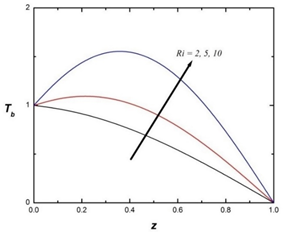Figure 2. Basic temperature distribution Tb vs. heat source parameter Ri

## 3a.png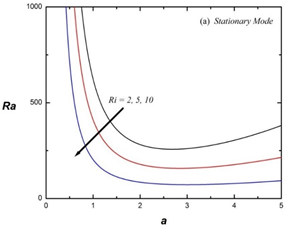## 3b.png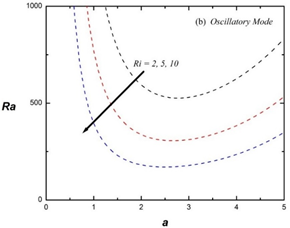Figure 3. Thermal Rayleigh number Ra vs. wave number a for distinct heat source parameter Ri

## 4a.png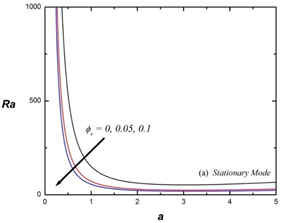## 4b.pngFigure 4. Thermal Rayleigh number Ra vs. wave number a for distinct volume fraction of nanoparticles ϕv

## 5a.png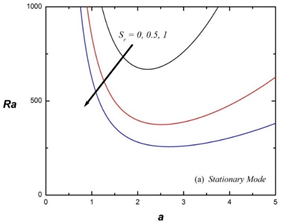## 5b.png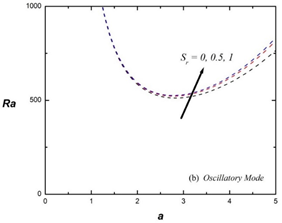Figure 5. Thermal Rayleigh number Ra vs. wave number a for distinct Soret parameter Sr

## 6a.png## 6b.pngFigure 6. Thermal Rayleigh number Ra vs. wave number a for distinct Dufour parameter Du

## 7a.png## 7b.pngFigure 7. Heat source parameter Ri’s effect on space (Rac, ψbf)

## 8a.png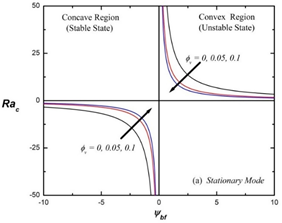## 8b.png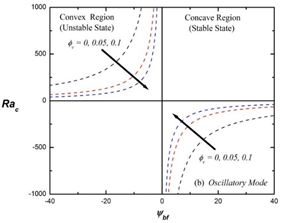Figure 8. Volume fraction of nanoparticles ϕvs effect on space (Rac, ψbf)

## 9a.png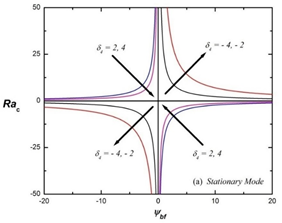## 9b.png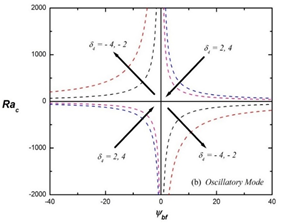Figure 9. Soret effect of nanoparticles δ4 on space (Rac, ψbf)

## 10a.png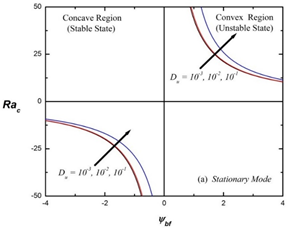## 10b.png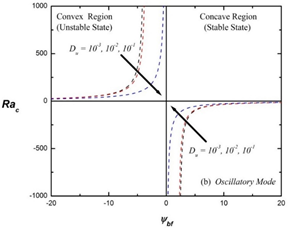Figure 10. Dufour parameter Du’s effect on space (Rac, ψbf)

## 11.png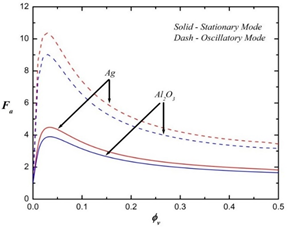Figure 11. Addition factor Fa vs. volume fraction of nanoparticles ϕv

The convective stability is expressed through the stability curves. The concave and convex regions of the curves represent the stable and unstable states of the system respectively. The neutral stability curves are depicted in Figures 3 – 6 for different values of heat source parameter Ri, volume fraction of nanoparticles ϕv, the Soret parameter Sr and the Dufour parameter Du respectively. From the Figure 3 it is observed that as Ri increases, the thermal Rayleigh number Ra decreases in both stationary (Figure 3a) and oscillatory (Figure 3b) states. Thus, internal heating shrinks the convective cells and causes to evolve the onset of convective instability in the system. Further from the Figures 4, 5 and 6, it is found that the Rayleigh number Ra decreases with an increase of volume fraction ϕv and the Soret parameter Sr and it increases with the Dufour parameter Du in stationary modes (Figures 4a, 5a, 6a). Hence, ϕv and Sr shrink the size of convection cells and Du enlarges the size of convection cells. If the size of convection cells is less, then the convective motion in the layer of binary nanofluid sets in easily and the layer is more unstable. Thus, volume fraction of nanoparticles ϕv and Soret parameter Sr have destabilizing effect, and Dufour parameter Du has stabilizing effect on the system. The opposite trend is noticed in case of oscillatory modes (Figures 4b, 5b, 6b).

Figures 7 and 8 are the graphs of critical Rayleigh number Rac versus separation ratio in binary basefluid ψbf for various internal heat source parameter Ri and volume fraction of nanoparticle ϕv respectively. In stationary mode (Figures 7a and 8a), it is observed that the critical Rayleigh number Rac decreases sharply with respect to absolute values of ψbf but in oscillatory mode (Figures 7b and 8b), it happens exactly reverse. It implies that the convective motion is produced easily with support of the separation ratio in binary basefluids i.e. Soret effect of solute ψbf. From the graphs, it has been realized that the stable state region is reduced and unstable state region is enlarged with an increase in the values of Ri and ϕv in both the modes of stationary and oscillatory convections. It signifies that the heat source parameter Ri and the volume fraction of nanoparticles ϕv have destabilizing effect on the system.

Soret effect of nanoparticles represented by the factor δ4 on the space (Rac, ψbf) is illustrated in Figure 9. For δ4 > 0, It is detected that the Rac decreases with an increase of δ4 and increases with respect to the Soret effect of solute ψbf. Thus, the thermodiffusion of nanoparticles strengthen the Brownian motion whereas thermodiffusion of solute weaken the motion in mode of stationary state (Figure 9a). Furthermore, it has been observed that the Rac decreases with an increase of δ4 with respect to ψbf in mode of oscillatory state. Here, thermodiffusion effects of both nanoparticles and solute strengthen the Brownian motion in binary nanofluid. The reverse effects have been noted for δ4 < 0. Through the above discussion, it is worked out that δ4 plays key role to decide the perfect pair of nanoparticle and solute for strengthening the convective heat transport in binary nanofluids in the presence of internal heat source.

Dufour effect represented by the factor Du on the Rayleigh number Rac with respect to separation ratio of basefluid ψbf is shown in Figure 10. In stationary mode (Figure 10a), the effect of Dufour parameter Du is insignificant on the stability states of the system i.e. there is no variation in the stability of the system, whereas its impact is considerable in case of oscillatory mode (Figure 10b). The stable state region becomes smaller and unstable state region becomes wider. Thus, the Dufour parameter helps to rise the onset of oscillatory convection in the system.

The profiles of addition factor Fa versus volume fraction ϕv are depicted in Figure 11. Due to lesser thermal conductivity of alumina than silver, the profile curves of addition factor Fa are always lie below in case of alumina based binary nanofluid of those in case of silver based binary nanofluid. Moreover, it is noticed that, the profiles of addition factor Fa increase up to particular value with an increase of volume fraction of nanoparticles then decrease gradually. It indicates that a little addition of nanoparticles in the basefluids causes to improve the transport of concentration and heat in binary nanofluids.

6. Conclusions

In the present work, investigation on the influence of internal heating with cross diffusions on convective stability of the system and heat transfer enhancement in binary nanofluids is made. From the above discussion and results, the following observations are summarized:

• The size of convection cells decreases with an increase in the values of internal Rayleigh number in both stationary and oscillatory states of the system.
• With an increase in the values of Dufour parameter, the size of convection cells increases and with volume fraction of nanoparticles and Soret coefficient, decreases in stationary mode. The effects are reversed in oscillatory mode.
• The internal heat source parameter i.e. internal Rayleigh number causes to increase the transfer of heat and concentration in the system.
• Stability of the system under the influence of cross diffusions depends upon the magnitudes of Soret effects of solute ψbf and nanoparticles δ4.
• Due to decrease in the addition factor Fa gradually in respect of volume fraction of nanoparticles ϕv causes to increase the heat transfer in binary nanofluid.

The results of the present study are useful to analyze the convective instability of binary nanofluids which are used as working fluids in many industrial applications such as absorption refrigeration and air conditioning, freezing of foods, solar collectors, medical treatment, electro and electroless plating, etc. They provide a good guideline to choose the best pair of nanoparticle and solute in binary nanofluids.

Acknowledgement

The authors acknowledge the University Grant Commission (UGC), Government of India, New Delhi for financial support under the scheme ‘Maulana Azad National Research Fellowship’. (F. No. 61-1/2019 (SA - III), dated 14/08/2019).

Nomenclature
 Latin symbols a dimensionless wave number C concentration, kg . m-3 CP heat capacity, W . kg-1 . K-1 h depth of nanofluid layer, m D solute diffusivity, m2 . s-1 Dsr diffusivity by Soret type Ddf diffusivity by Dufour type D differential operator (= d/dz) Du Dufour parameter Fa addition factor g gravitational acceleration, m2 . s-1 k thermal conductivity, W . m-3 . K-1 Le Lewis number P pressure, Pa Pr Prandtl number Q amount of heat flux Ra thermal Rayleigh number RaS solute Rayleigh number Ri internal Rayleigh number So solutal parameter Sr Soret parameter T temperature, K $\bar{q}$ velocity vector, m . s-1 Ve effective volume of nanoparticles W vertical velocity Greek letters α thermal diffusivity, m2 . s-1 βS solute expansion βT thermal expansion μ dynamic viscosity ν kinetic viscosity ρ density, kg . m-3 ϕv volume fraction of nanoparticles ψ separation ratio ($D \beta_{s} / D_{s r} \beta_{T}$) ω frequency of oscillations Subscripts b basic state bf binary basefluid nf nanofluid np nanoparticle s solute quantity 0 reference position Other symbols ${{\nabla }^{2}}\,\,=\,\,\frac{{{\partial }^{2}}}{\partial {{x}^{2}}}\,+\,\frac{{{\partial }^{2}}}{\partial {{y}^{2}}}+\,\frac{{{\partial }^{2}}}{\partial {{z}^{2}}}$ $\nabla _{1}^{2}\,\,=\,\,{{\nabla }^{2}}\,-\,\,\frac{{{\partial }^{2}}}{\partial {{z}^{2}}}$

Appendix

\begin{align} & {{\Delta }_{1}}=\,\,\frac{{{A}_{1}}{{B}_{6}}\,{{\omega }^{2}}+{{A}_{2}}{{B}_{7}}}{{{B}_{4}}\,{{\omega }^{2}}+{{B}_{5}}} \\ & {{\Delta }_{2}}=\,\,\frac{{{A}_{2}}{{B}_{6}}-{{A}_{1}}{{B}_{7}}}{{{B}_{4}}\,{{\omega }^{2}}+{{B}_{5}}} \\ & {{A}_{1}}=\,\,2F{{\delta }^{2}}(1+{{S}_{r}})-{{\delta }^{2}}{{D}_{u}}-{{S}_{o}}({{\delta }^{2}}+Ri) \\ & {{A}_{2}}=2F\,Le\,-{{S}_{o}} \\ & {{A}_{3}}=\,\,{{\delta }^{2}}(1-K)+Ri \\ & {{A}_{4}}\,\,=\,\,{{\delta }^{2}}Le+\frac{{{\delta }^{2}}}{\Pr }+\frac{{{\delta }^{2}}Le\,}{\Pr }+\frac{Le\,Ri}{\Pr } \\ & {{A}_{5}}\,\,=\,{{\Pr }^{-1}}Le \\ & {{A}_{6}}=\,\,{{\delta }^{2}}+\,Le\,\left( {{\delta }^{2}}+\,Ri \right)\,\,+\,\,\frac{{{A}_{3}}}{\Pr } \\ & {{B}_{1}}=\,\,{{A}_{1}}{{A}_{5}} \\ & {{B}_{2}}=\,{{A}_{1}}\,{{A}_{6}}-{{A}_{2}}{{A}_{4}} \\ & {{B}_{3}}=\,\,{{A}_{2}}{{A}_{3}} \\ & {{B}_{4}}=\,\,A_{1}^{2} \\ & {{B}_{5}}=\,\,A_{2}^{2} \\ & {{B}_{6}}=\,\,{{\delta }^{2}}\left( {{B}_{1}}-{{\omega }^{2}}Le \right) \\ & {{B}_{7}}=\,\,{{\delta }^{4}}{{B}_{6}}-{{\omega }^{2}}{{B}_{5}} \\\end{align}

References

 Das, S.K., Choi, S.U.S., Yu, W., Pradeep, T. (2007). Nanofluids: Science and Technology. John Wiley and Sons, Inc., Hoboken, New Jersey, pp. 337-352. https://doi.org/10.1002/9780470180693

 Wang, L., Quintard, M. (2009). Nanofluids of the Future. In: Wang L. (eds) Advances in Transport Phenomena. Advances in Transport Phenomena, vol 1. Springer, Berlin, Heidelberg. https://doi.org/10.1007/978-3-642-02690-4_4

 Choi, S., Eastman, J.A. (1995). Enhancing Thermal Conductivity of Fluids with Nanoparticles. ASME Fluids Engineering Division, 231: 99-105.

 Ramanathan, A., Gunasekaran, P. (2008). Simulation of absorption refrigeration system for automobile application. Thermal Sciences, 12(3): 5-13. https://doi.org/10.2298/TSCI0803005R

 Kuznetsov, I.A., Greenfield, M.J., Mehta, Y.V., Merchan, W., Salkar, G., Saveliev, A.V. (2011). Increasing the solar cell power output by coating with transition metal oxide nanorods. Applied Energy, 88(11): 4218-4221. https://doi.org/10.1016/j.apenergy.2011.04.033

 Singh, R.P., Heldman, D.R. (2014). Introduction to Food Engineering. 4th ed. Academic Press, San Diego, California, USA. https://doi.org/10.1016/C2011-0-06101-X

 Kim, J.K., Jung, J.Y., Kang, Y.T. (2007). Absorption performance enhancement by nanoparticles and chemical surfactants in binary nanofluids. International Journal of Refrigeration, 30(1): 50-57. https://doi.org/10.1016/j.ijrefrig.2006.04.006

 Ma, X., Su, F., Chen, J., Zhang, Y. (2007). Heat and mass transfer enhancement of the bubble absorption for a binary nanofluid. Journal of Mechanical Science and Technology, 21: 1813-1818. https://doi.org/10.1007/BF03177437

 Lee, J.K., Koo, J., Hong, H., Kang, Y.T. (2010). The effect of nanoparticles on absorption heat and mass transfer performance in NH3 / H2O binary nanofluid. International Journal of Refrigeration, 33(2): 269-275. https://doi.org/10.1016/j.ijrefrig.2009.10.004

 Pang, C., Wu, W., Sheng, W., Zhang, H., Kang, Y.T. (2012). Mass transfer enhancement by binary nanofluid (NH3/H2O + Ag Nanoparticles) for bubble absorption process. International Journal of Refrigeration, 35(8): 2240-2247. http://dx.doi.org/10.1016/j.ijrefrig.2012.08.006

 Xuan, Y. (2009). Conception for enhanced mass transport in binary nanofluids. Heat and Mass Transfer, 46: 277-279. https://doi.org/10.1007/s00231-009-0564-z

 Chand, R., Rana, G.C. (2012). On the onset of thermal convection in rotating nanofluid layer saturated Darcy-Brinkman model. International Journal of Heat and Mass Transfer, 55(21-22): 5417-5424. http://dx.doi.org/10.1016/j.ijheatmasstransfer.2012.04.043

 Chand, R., Rana, G.C. (2012). Thermal instability of Rivlin - Ericksen elastic-viscous nanofluid saturated by porous medium. Journal of Fluid Engineering, 134(12): 1-7. https://doi.org/10.1115/1.4007901

 Buongiorno, J. (2005). Convective transport in nanofluids. Journal of Heat and Mass Transfer, 128(3): 240-250. https://doi.org/10.1115/1.2150834

 Nield, D.A., Kuznetsov, A.V. (2010). The onset of double diffusive nanofluid convection in a layer of a saturated porous medium. Transport in Porous Media, 85: 941-951. https://doi.org/10.1007/s11242-010-9600-1

 Yadav, D., Agarwal, G.S., Bhargava, R. (2012). The onset of convection in a binary nanofluid saturated porous layer. International Journal of Theoretical and Applied Multiscale Mechanics, 2(3): 198-224. https://doi.org/10.1504/IJTAMM.2012.049931

 Bhadauria, B.S., Agarwal, S., Sachetil, N.C., Chandran, P., Singh, A.K. (2012). Nonlinear convective transport in a binary nanofluid saturated porous layer. Transport in Porous Media, 93: 29-49. https://doi.org/10.1007/s11242-012-9942-y

 Gupta, U., Sharma, J., Sharma, V. (2015). Instability of binary nanofluid with magnetic field. Applied Mathematics and Mechanics, 36(6): 693-706. https://doi.org/10.1007/s10483-015-1941-6

 Kim, J., Kang, Y.T., Choi, C.K. (2003). Analysis of convective instability and heat transfer characteristics of nanofluids. Physics of Fluids, 16: 2395-2401. https://doi.org/10.1063/1.1739247

 Kim, J., Kang, Y.T., Choi, C.K., Kim, M.G. (2005). Effects of thermodiffusion and nanoparticles on convective instabilities in binary nanofluids. Journal of Nanoscale and Microscale Thermophysical Engineering, 10(1): 29-39. https://doi.org/10.1080/10893950500357772

 Kim, J., Kang, Y.T., Choi, C.K. (2007). Soret and Dufour effects on convective instabilities in binary nanofluids for absorption application. International Journal of Refrigeration, 30: 323-328. https://doi.org/10.1016/j.ijrefrig.2006.04.005

 Gaikwad, S.N., Kouser, S. (2014). Double diffusive convection in couple stress fluid saturated porous layer with internal heat source. International Journal of Heat and Mass Transfer, 78: 1254-1264. https://doi.org/10.1016/j.ijheatmasstransfer.2014.07.021

 Yadav, D., Wang, J. (2018). Convective heat transport in a heat generating porous layer saturated by a Non-Newtonian nanofluid. Heat Transfer Engineering, 40(16): 1363-1382. https://doi.org/10.1080/01457632.2018.1470298

 Yadav, D., Bhargava, R., Agrawal, G.S. (2012). Effect of internal heat source on the onset of convection in nanofluid layer. Applied Mechanics of Materials, 110-116: 1827-1832. https://doi.org/10.4028/www.scientific.net/AMM.110-116.1827

 Nield, D.A., Kuznetsov, A.V. (2013). The onset of convection in an internally heated nanofluid layer. Journal of Heat Transfer, ASME Series, 136(1): 014501.

 Kanchana C., Zhao, Y. (2018). Effect of internal heat generation/absorption on Rayleigh - Benard convection in water well-dispersed with nanoparticles or carbon nanotubes. International Journal of Heat and Mass Transfer, 127: 1031-1047. https://doi.org/10.1016/j.ijheatmasstransfer.2018.06.12

 Gaikwad, S.N., Surwase, D.M. (2020). Convective instability in binary nanofluids with an internal heat source in the presence of thermodiffusion and nanoparticles. Heat Transfer, 49(8): 4511-4527. https://doi.org/10.1002/htj.21838

 Hort, W., Linz, S.J., Lucke, M. (1992). Onset of convection in binary gas mixtures: Role of the Dufour effect. Physics Review A, 45(6): 3737-3748. https://doi.org/10.1103/PhysRevA.45.3737

 Drazin, P.G., Reid, W.H. (1981). Hydrodynamics Stability. Cambridge University Press, London.

 Chandrasekhar, S. (1961). Hydrodynamic and Hydromagnetic Stability. Oxford University Press, London.

 Cengel, Y.A. (1998). Heat Transfer: A Practical Approach. WCB/McGraw Hill, New York.

 Razif, N.H.M., Mamata, A.M.I.B., Lias, I., Mohamed, W.A.N.W. (2015) Thermophysical properties analysis for ammonia - water mixture of an organic Rankine cycle. Journal Teknologi (Science and Engineering), 75(8): 13-17. https://doi.org/10.11113/jt.v75.5203